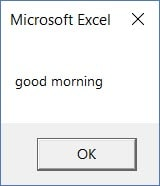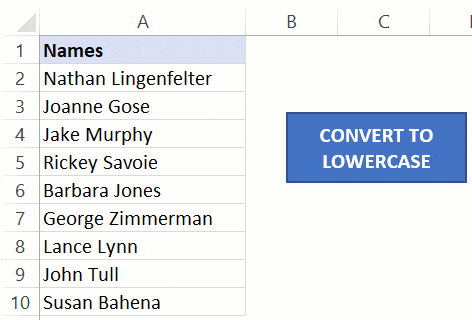The VBA LCASE function takes a string as the input and converts it into a lower case string - EBOOK VBA EXCELEVBA.info ( Ebook VBA Excel ) Welcome EVBA.info - Nothing Is Unable ... About Excel Tricks, Learning VBA Programming, Dedicated Software, Free Courses, Living Skills ...

## Wednesday, January 15, 2020

In Excel worksheet, there is a LOWER function that converts a text string into lower case.
Just like the LOWER function, there is a similar inbuilt function in Excel VBA – LCASE
The VBA LCASE function takes a string as the input and converts it into a lower case string.

## Syntax of VBA LCASE Function

Below is the syntax of the VBA LCase function
`LCase(String)`
‘String’ is the text that you want to convert to lower case.
You can use a text string, a range reference that contains the text string, or a variable that has the text string.
Let’s have a look at a couple of example of using the LCase function.

## VBA LCase Examples

The below code would convert the specified text into lower case and show it in a message box.
```Sub LCaseExample1()
MsgBox LCase("Good Morning")
End Sub```Here is another example, where we have used a variable (‘Var’) to hold the text string and then used the LCase function to convert it into lower case.
```Sub LCaseExample2()
Dim Var As String
Var = "Good Morning"
MsgBox LCase(Var)
End Sub```
Another example below shows how to take the string from a cell (A1) and show the lowercase version of it in a message box.
```Sub LCaseExample3()
MsgBox LCase(Range("A1"))
End Sub```
While all these above examples work, you’re unlikely to use this function to simply convert or show the lowercase string.
Below is a more practical example of the LCase function in Excel VBA. The below code would go through all the cells in the selected range and convert all the text strings into lower case.
```Sub LCaseExample1()
Dim rng As Range
Set rng = Selection
For Each Cell In rng
Cell.Value = LCase(Cell)
Next Cell
End Sub```A few important things to know about the VBA LCase function:
1. It affects only the Uppercase characters of the text string. Any character other than the Uppercase text characters is left unchanged.
2. If you use a null character (or a reference to an empty cell), it will return a null character.
#evba #etipfree #kingexcel
📤You download App EVBA.info installed directly on the latest phone here : https://www.evba.info/p/app-evbainfo-setting-for-your-phone.html?m=1